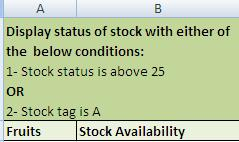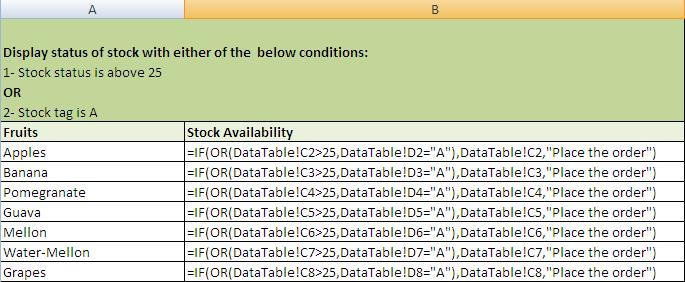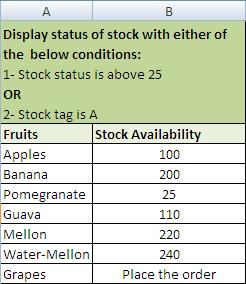# IF OR combined Function MS Excel

## Step by Step: How to Use ‘IF / OR’ Combined in a single formula in excel 2003, 2007 & 2010

IF OR combined Function MS Excel: What is the Use of IF…OR in combination of IF Condition? Generally the IF() formula is used to specify a single condition and certain other calculations can be derived by using the combined formula of IF…OR. Simple IF is displayed to show you the limitations of an IF condition:

a) =IF(A1=100, Value if true, values if false)

The description of IF…OR function is shown as below:

b) IF condition with And: =IF(OR(A1=100,B1>50,C1<10),Value if True, Value if False)
The OR function has been italicized for clarity of use and OR(A1=100,B1>50,C1<10) shows the optional and alternative use of the conditions. If any of these conditions are met, the formula shall perform.

A detailed use of IF…OR Function in MS Excel 2007, 2010 is displayed below step by step:

### Step 1 for IF OR in MS Excel:

(b) Formulate your tables: these may be on the same table or on a different worksheet or even different workbooks.
(c) For the sake of example we have created two Tables.
(d) Table from where data is to be retrieved:IF…OR condition combined MS excel-1

(e) Table where data is to be fetched/desired.MS excel using IF OR

### Step 2: for IF OR in MS Excel

Ensure spellings for search values are the same in both the tables.

### Step 4: Step by step checking the final formula of IF OR in combinationStep by step MS excel using IF OR

### Final Formula: MS Excel IF and ORMS excel If OR function combined Fomula

### Result: MS Excel FunctionMS Excel IF OR Function Combined-2